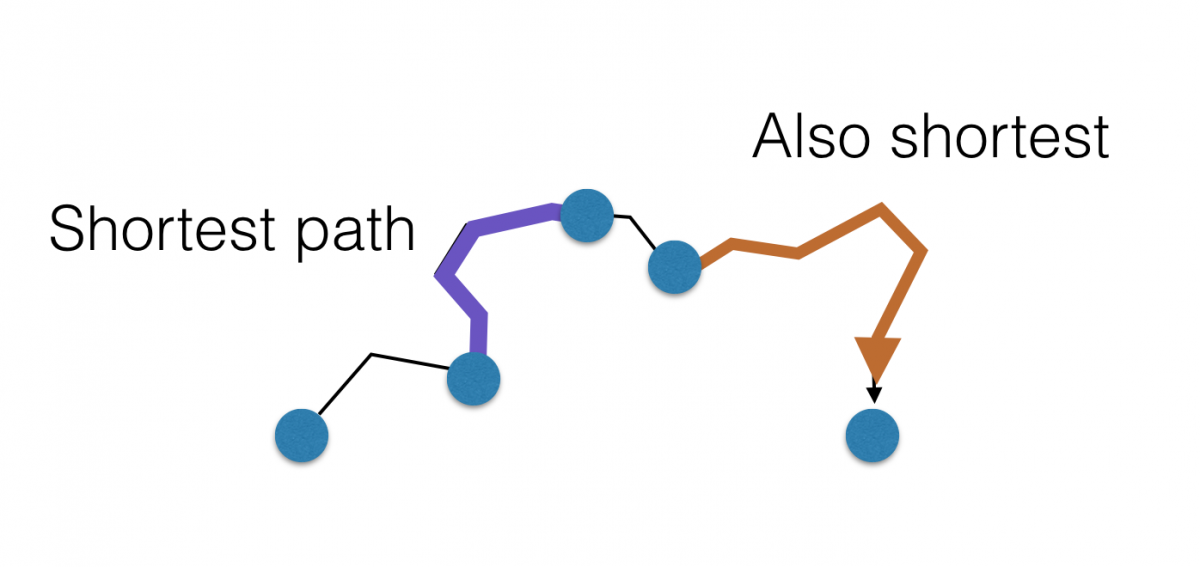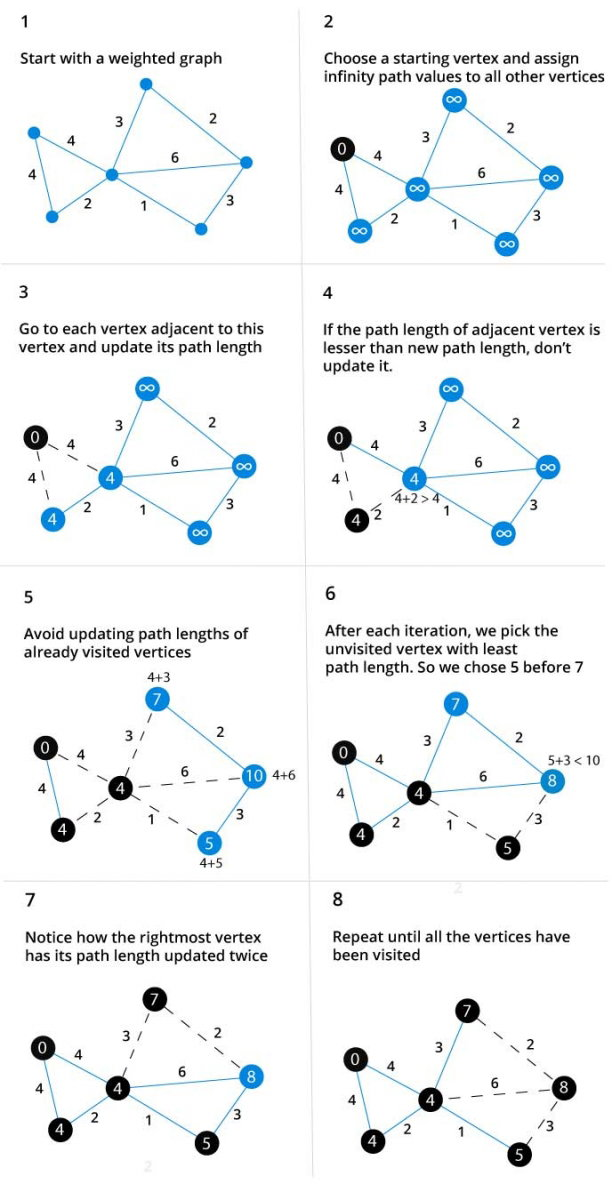Dijkstra's Algorithm

Dijkstra's algorithm allows us to find the shortest path between any two vertices of a graph.

It differs from minimum spanning tree because the shortest distance between two vertices might not include all the vertices of the graph.

How Dijkstra's Algorithm works

Dijkstra's Algorithm works on the basis that any subpath B -> D of the shortest path A -> D between vertices A and D is also the shortest path between vertices B and D.Djikstra used this property in the opposite direction i.e we overestimate the distance of each vertex from the starting vertex. Then we visit each node and its neighbours to find the shortest subpath to those neighbours.

The algorithm uses a greedy approach in the sense that we find the next best solution hoping that the end result is the best solution for the whole problem.

Example of Dijkstra's algorithm

It is easier to start with an example and then think about the algorithm.Djikstra's algorithm pseudocode

We need to maintain the path distance of every vertex. We can store that in an array of size v, where v is the number of vertices.

We also want to able to get the shortest path, not only know the length of the shortest path. For this, we map each vertex to the vertex that last updated its path length.

Once the algorithm is over, we can backtrack from the destination vertex to the source vertex to find the path.

A minimum priority queue can be used to efficiently receive the vertex with least path distance.

function dijkstra(G, S)
for each vertex V in G
distance[V] <- infinite
previous[V] <- NULL
If V != S, add V to Priority Queue Q
distance[S] <- 0

while Q IS NOT EMPTY
U <- Extract MIN from Q
for each unvisited neighbour V of U
tempDistance <- distance[U] + edge_weight(U, V)
if tempDistance < distance[V]
distance[V] <- tempDistance
previous[V] <- U
return distance[], previous[]

Code for Dijkstra's Algorithm

The implementation of Dijkstra's Algorithm in C++ is given below. The complexity of the code can be improved, but the abstractions are convenient to relate the code with the algorithm.

#include <iostream>
#include <vector>

#define INT_MAX 10000000

using namespace std;

void DijkstrasTest();

int main()
{
DijkstrasTest();
return 0;
}

class Node;
class Edge;

void Dijkstras();
Node* ExtractSmallest(vector<Node*>& nodes);
int Distance(Node* node1, Node* node2);
bool Contains(vector<Node*>& nodes, Node* node);
void PrintShortestRouteTo(Node* destination);

vector<Node*> nodes;
vector<Edge*> edges;

class Node
{
public:
Node(char id)
: id(id), previous(NULL), distanceFromStart(INT_MAX)
{
nodes.push_back(this);
}
public:
char id;
Node* previous;
int distanceFromStart;
};

class Edge
{
public:
Edge(Node* node1, Node* node2, int distance)
: node1(node1), node2(node2), distance(distance)
{
edges.push_back(this);
}
bool Connects(Node* node1, Node* node2)
{
return (
(node1 == this->node1 &&
node2 == this->node2) ||
(node1 == this->node2 &&
node2 == this->node1));
}
public:
Node* node1;
Node* node2;
int distance;
};

///////////////////
void DijkstrasTest()
{
Node* a = new Node('a');
Node* b = new Node('b');
Node* c = new Node('c');
Node* d = new Node('d');
Node* e = new Node('e');
Node* f = new Node('f');
Node* g = new Node('g');

Edge* e1 = new Edge(a, c, 1);
Edge* e2 = new Edge(a, d, 2);
Edge* e3 = new Edge(b, c, 2);
Edge* e4 = new Edge(c, d, 1);
Edge* e5 = new Edge(b, f, 3);
Edge* e6 = new Edge(c, e, 3);
Edge* e7 = new Edge(e, f, 2);
Edge* e8 = new Edge(d, g, 1);
Edge* e9 = new Edge(g, f, 1);

a->distanceFromStart = 0; // set start node
Dijkstras();
PrintShortestRouteTo(f);
}

///////////////////

void Dijkstras()
{
while (nodes.size() > 0)
{
Node* smallest = ExtractSmallest(nodes);

const int size = adjacentNodes->size();
for (int i=0; i<size; ++i)
{
int distance = Distance(smallest, adjacent) +
smallest->distanceFromStart;

if (distance < adjacent->distanceFromStart)
{
}
}
}
}

// Find the node with the smallest distance,
// remove it, and return it.
Node* ExtractSmallest(vector<Node*>& nodes)
{
int size = nodes.size();
if (size == 0) return NULL;
int smallestPosition = 0;
Node* smallest = nodes.at(0);
for (int i=1; i<size; ++i)
{
Node* current = nodes.at(i);
if (current->distanceFromStart <
smallest->distanceFromStart)
{
smallest = current;
smallestPosition = i;
}
}
nodes.erase(nodes.begin() + smallestPosition);
return smallest;
}

// Return all nodes adjacent to 'node' which are still
// in the 'nodes' collection.
{
vector<Node*>* adjacentNodes = new vector<Node*>();
const int size = edges.size();
for(int i=0; i<size; ++i)
{
Edge* edge = edges.at(i);
Node* adjacent = NULL;
if (edge->node1 == node)
{
}
else if (edge->node2 == node)
{
}
{
}
}
}

// Return distance between two connected nodes
int Distance(Node* node1, Node* node2)
{
const int size = edges.size();
for(int i=0; i<size; ++i)
{
Edge* edge = edges.at(i);
if (edge->Connects(node1, node2))
{
return edge->distance;
}
}
return -1; // should never happen
}

// Does the 'nodes' vector contain 'node'
bool Contains(vector<Node*>& nodes, Node* node)
{
const int size = nodes.size();
for(int i=0; i<size; ++i)
{
if (node == nodes.at(i))
{
return true;
}
}
return false;
}

///////////////////

void PrintShortestRouteTo(Node* destination)
{
Node* previous = destination;
cout << "Distance from start: "
<< destination->distanceFromStart << endl;
while (previous)
{
cout << previous->id << " ";
previous = previous->previous;
}
cout << endl;
}

// these two not needed
vector<Edge*>* AdjacentEdges(vector<Edge*>& Edges, Node* node);
void RemoveEdge(vector<Edge*>& Edges, Edge* edge);

vector<Edge*>* AdjacentEdges(vector<Edge*>& edges, Node* node)
{
vector<Edge*>* adjacentEdges = new vector<Edge*>();

const int size = edges.size();
for(int i=0; i<size; ++i)
{
Edge* edge = edges.at(i);
if (edge->node1 == node)
{
cout << "adjacent: " << edge->node2->id << endl;
}
else if (edge->node2 == node)
{
cout << "adjacent: " << edge->node1->id << endl;
}
}
}

void RemoveEdge(vector<Edge*>& edges, Edge* edge)
{
vector<Edge*>::iterator it;
for (it=edges.begin(); it<edges.end(); ++it)
{
if (*it == edge)
{
edges.erase(it);
return;
}
}
}